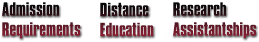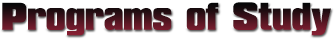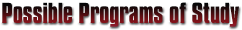We take the time to customize your curriculum by tailoring the course offerings to match your computational experience, foundation and future career goals. The MSU CME graduate program features many accomplished academic leaders who are eager to share their extensive range of expertise and insights gained from successful research accomplishments and enlightened experiences.The programs of study listed below are for illustration purposes only! Actual programs of study are strongly influenced by each student's background in and pre-requisite knowledge of the three areas of emphasis: high-performance computing,numerical mathematics and the engineering application area. Each program of study is customized to the needs of the individual student, and is finalized with the assistance of the CME coordinator, the student's major professor, and the student's graduate committee.

##### M.S. Program of Study, Thesis Option
Course Credits Title Notes
M.A. 8443 3 Numerical Solution of Partial Differential Equations I Numerical math
M.A. 8453 3 Numerical Solution of Partial Differential Equations II Numerical math
CSE 6753 3 Foundations in Computation High-performance computing
CSE 6163 3 Designing Parallel Algorithms High-performance computing
CSE 6833 3 Introduction to Analysis of Algorithms High-performance computing
ASE 6423 3 Introduction to Computational Fluid Dynamics Application area
ASE 8413 3 Computational Fluid Dynamics I Application area
ASE 8423 3 Computational Fluid Dynamics II Application area
CME 8000 6 Thesis research

Total: 30 credits Tailored to a student with an Aerospace or Mechanical Engineering background, interested in Computational Fluid Dynamics
##### M.S. Program of Study, Non-thesis Option
Course Credits Title Notes
M.A. 6313 3 Numerical Analysis I Numerical math
M.A. 6323 3 Numerical Analysis II Numerical math
M.A. 8363 3 Numerical Solution of Systems of Nonlinear Equations Numerical math
M.A. 8383 3 Numerical Solution of Ordinary Differential Equations I Numerical math
CSE 6753 3 Foundations in Computation High-performance computing
CSE 6163 3 Designing Parallel Algorithms High-performance computing
ECE 6713 3 Computer Architecture High-performance computing
CHE 8113 3 Advanced Chemical Engineering Thermodynamics Application area
CHE 8123 3 Chemical Kinetics and Dynamics Application area
CHE 8223 3 Advanced Process Computations Application area
CME 7000 3 Directed Individual Study Project

Total: 33 credits Tailored to a student with a Chemical Engineering background, interested in high-fidelity simulations of chemical processes
##### Ph.D. Program of Study, Regular Admission
Course Credits Title Notes
M.A. 8203 3 Foundations of Applied Mathematics I Numerical math
M.A. 8213 3 Foundations of Applied Mathematics II Numerical math
M.A. 8463 3 Numerical Linear Algebra Numerical math
CSE 6753 3 Foundations in Computation High-performance computing
CSE 6163 3 Designing Parallel Algorithms High-performance computing
CSE 6214 4 Software Engineering High-performance computing
IE 6773 3 System Simulation I Application area
IE 8773 3 System Simulation II Application area
CME 9000 25 Dissertation Research

Total: 50 credits Student already has a M.S. degree in Industrial Engineering.
##### Ph.D. Program of Study, Direct Admission
Course Credits Title Notes
M.A. 8363 3 Numerical Solution of Systems of Nonlinear Equations Numerical math
M.A. 8443 3 Numerical Solution of Partial Differential Equations I Numerical math
M.A. 8453 3 Numerical Solution of Partial Differential Equations II Numerical math
M.A. 8383 3 Numerical Solution of Ordinary Differential Equations I Numerical math
M.A. 8393 3 Numerical Solution of Ordinary Differential Equations I Numerical math
CSE 6753 3 Foundations in Computation High-performance computing
CSE 6163 3 Designing Parallel Algorithms High-performance computing
CSE 6833 3 Introduction to Analysis of Algorithms High-performance computing
CSE 8833 3 Algorithms High-performance computing
CSE 6413 3 Principles of Computer Graphics Graphics and Visualization
CSE 8413 3 Visualization Graphics and Visualization
ECE 6413 3 Digital Signal Processing Graphics and Visualization
ECE 8443 3 Pattern Recognition Application area
ECE 8453 3 Intro to Wavelets Application area
ECE 8473 3 Digital Image Processing Application area
ECE 8483 3 Image and Video Coding Application area
CME 9000 24 Dissertation Research

Total: 72 credits Student admitted with only a BS degree in math, physics, or engineering.